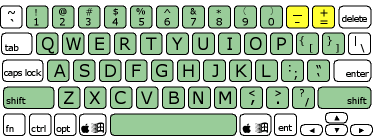Ok, so strictly speaking they're not exclusively math keys, but it is useful to have some way reference them. It is helpful to remember that these simple arithmetic operators reside in the very top row, alongside the numbers.

Both keys are accessed with the right-hand pinky finger in an extended position.

Before trying the exercise, try running the Math Key Pinky Buster a number of times to get used to moving your pinky finger from the home row all the way to the math keys -- it's quite a distance!

You wouldn't dare start this exercise without reminding yourself of the Principles for Effective Learning, would you?

-++- -_-_ +_-_ =_=+ __+ -= =+ -=_ +-_- =+++ + ++_ ++= =+= ++- +-- -+++ =-- +- -= -+= _++ --+ =+ -_+ +=_ -- +_ +-_ -+-- ___ _+ =+_= - -+= = + =__ -=+ =+_ =-= ++ += =++ =_+_ -__